# SBI PO Reasoning Questions 2019 (Day-14) High Level-New Pattern

SBI PO 2019 Notification is about to come and it is the most awaited exam among the aspirants. We all know that new pattern questions are introducing every year in the SBI PO exam. Further, the questions are getting tougher and beyond the level of the candidate’s expectations.

Our IBPS Guide is providing High-Level New Pattern Reasoning Ability Questions for SBI PO 2019 so the aspirants can practice it on a daily basis. These questions are framed by our skilled experts after understanding your needs thoroughly. Aspirants can practice these high-level questions daily to familiarize with the exact exam pattern. We wish that your rigorous preparation leads you to a successful target of becoming SBI PO.

[WpProQuiz 5053]

### Click Here for SBI PO Pre 2019 High-Quality Mocks Exactly on SBI Standard

Directions (1-5): Study the following information carefully and answer the below questions.

Ten person namely – Mayank, Rinku, Bittu, Payal, Ishu, Dev, Kavita, Hari, Sam and Anuj are sitting around a rectangular table in such a way that one person sits on each smaller side, two persons on each bigger side and one person at each corners. The persons at the four sides sits facing center while persons at the four corners sits facing away from center. Weight of each person is different viz. 42, 48, 52, 56, 64, 68, 72, 76, 78 and 84 kg. All the information is not necessary to be in the same order.

Numbers in the given figure represents their respective position.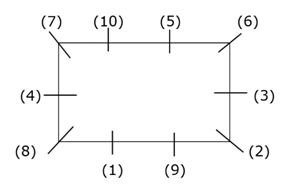The one whose weight is 84 kg sits at longer side at a gap of two places from Anuj, whose weight is neither 72 kg nor 64 kg. The one whose weight is 52 kg sits second to left of Rinku. One person sits between the one whose weight is 48 kg and the one whose weight is 68 kg. Three people sits between Anuj and the one whose weight is 48 kg, who neither sits adjacent to Payal nor sits adjacent to the one whose weight is 84 kg. The one whose weight is 76kg sits facing Ishu, who neither sits adjacent to Sam nor the one whose weight is 82kg. The one whose weight is 68 kg and Kavita sits together at longer side. One person sits between Ishu and the one whose weight is 72kg. Either Bittu or Hari sits at corner of the table. Payal doesn’t sit adjacent to the one whose weight is 48 kg. Two people sit between Bittu and Hari, who sits adjacent to the one whose weight is 64 kg. Two people sit between Kavita and Dev whose weight is 56 kg. The one whose weight is 52 kg sits third to the left of Payal, whose weight is 42 Kg.

Now they start playing card game. The shuffled a pack of card, each person draws one card and change their position according to given conditions:

Condition 1: If the card drawn is a face card of ‘Diamond’ then, the person who draws card interchange his/her position with the one who sits at position 2, else interchange his/her position with the one who sits at position 5.

Condition 2: If the card drawn is a number card of ‘Club’ then, the person who draws card interchange his/her position with the one who sits at position 6, else interchange his/her position with the one who sits at position 4.

Condition 3: If the card drawn is a face card of ‘Heart’ then, the person who draws card interchange his/her position with the one who sits at position 7, else interchange his/her position with the one who sits at position 10.

Condition 4: If the card drawn is a number card of ‘Spade’ then, the person who draws card interchange his/her position with the one who sits at position 1, else interchange his/her position with the one who sits at position 3.

Note:- All the person draws cards in same sequence as given below:

i) Mayank draws 7 of diamond.

ii) Rinku draws King of heart.

iii) Payal draws Queen of club.

iv) Anuj draws 10 of club.

v) Kavita draws ace of spade.

vi) Hari draws King of club.

vii) Sam draws 5 of spade.

viii) Dev draws ace of diamond.

ix) Ishu draws 4 of heart.

x) Bittu draws 9 of spade.

1) What is the position of Bittu with respect to Sam in the final arrangement?

a) Third to left

b) Fourth to right

c) Immediate left

d) Second to right

e) None of the above.

2) How many people remain unchanged after final arrangement?

a) One

b) Three

c) Two

d) None

e) Four

3) How many person sits between the one whose age is 64 years and the one whose age is 52 years when counted right of the one whose age is 64 years after final arrangement?

a) Four

b) Two

c) One

d) Five

e) None

4) Who sits to the immediate right of Rinku before playing cards?

a) Ishu

b) Hari

c) Payal

d) Anuj

e) None of the above

5) What is the position of Dev with respect to Mayank before playing cards?

a) Third to left

b) Fourth to right

c) Immediate left

d) Second to right

e) None of the above.

Directions (6-10): Study the following information to answer the given questions.

A, B, C and D are the corner points of the rectangular field in such a way that A is west of point B. C is south of point A. Side CD is the largest side of the field. Point D is 18m north of point I. E, F, G and H are the corner points of another rectangular field having area of 2304 m2 in such a way that Line EF intersects Line AB at M and Line CD at N respectively. Point E is North of F. C is 12m west of point N. The length of largest side of field ABCD is 80m.Point I is 54m south of point B. H is 32m east of point E. Line GH intersects the rectangular field at L and P. D, I, J and K form another rectangular field in such a way that point J is 8m east of point P. Area of the rectangular field DIJK is 504m2. Point H is 8m from point L.

Note: Area of rectangle= length * breadth (m2)

6) What is position of K with respect to G?

a) North-East, 2√41m

b) South-West, 11√2m

c) East, 12m

d) North-East, 4√13m

e) East, 8m

7) If Sam standing at point F, wants to reach point B, then which of the following is the correct shortest direction to reach his destination?

a) FGPDB

b) FNCMB

c) FGLB

d) FEHLB

e) Either A or C

8) What is the difference of area formed by MLPN to EHLM?

a) 768m2.

b) 752m2.

c) 642m2.

d) 392m2.

e) None of these

9) What is the shortest distance between M and H?

a) 2√58m

b) 3√62m

c) 4√68m

d) 3√68 m

e) 5√60m

10) In which direction is Point B with respect to N?

a) South-east

b) North-east

c) East

d) North-west

e) North

Directions (1-5):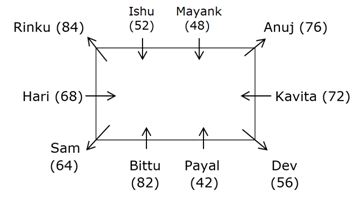We have:

• The one whose weight is 84 kg sits at longer side at a gap of two place from Anuj, that means we have four possibility for Anuj, in case (1a) & case (2b) Anuj sits at corner, in case (1b) & case (2a) Anuj sits at smaller side.
• Three people sits between Anuj and the one whose weight is 48 kg, who neither sits adjacent to Payal nor sits adjacent to the one whose weight is 84 kg.
• One person sits between the one whose weight is 48 kg and the one whose weight is 68 kg.
• The one whose weight is 68 kg and Kavita sits together at longer side, that means we have six possibility for Kavita, in case (1a) Kavita sits immediate right of Anuj, in case (1b) Kavita sits immediate right of the one whose weight of 48 kg, in case (1c)& case (2c) weight of Kavita is 84 kg, in case (2a) Kavita sits third to left of Anuj, in case (2b) Kavita sits immediate left of Anuj.
• Two person sits between Kavita and Dev whose weight is 56 kg, that means in case (1a) & case (1b) Dev sits immediate left of the one whose weight is 84 kg, in case (2a) & case (2b) Dev sits immediate right of the one whose weight is 84 kg and case (1c) & case (2c) are not valid.

Based on above given information we have: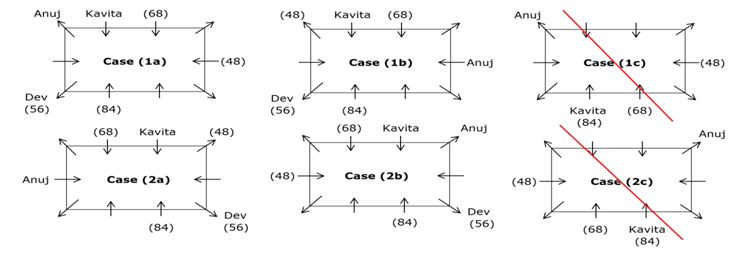Case (1c) & case (2c) are not valid as two person sits between Kavita and Dev whose weight is 56kg.

Again, we have:

• The one whose weight is 52 kg sits third to left of Payal, whose weight is 42 Kg.
• The one whose weight is 52 kg sits second to left of Rinku.
• As Payal doesn’t sit adjacent to the one whose weight is 48 kg, that means in case (1a) & case (1b) Payal sits immediate right of the one whose weight is 84 kg and case (2a) & case (2b) are not valid.
• Two people sit between Bittu and Hari, who sits adjacent to the one whose weight is 64 kg.
• Either Bittu or Hari sits at corner of the table, that means Bittu sits at corner and Hari sits immediate left of the one whose weight is 64 kg.

Based on above given information we have: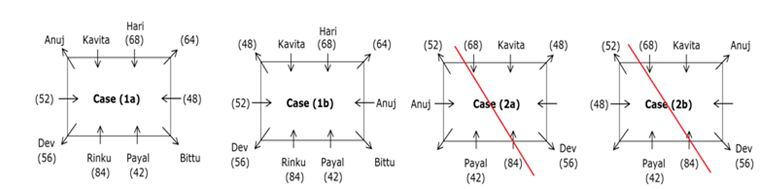Case (2a) & case (2b) are not valid as the one whose weight is 52 kg sits second to left of Rinku.

Again, we have:

• The one whose weight is 76kg sits facing Ishu, who neither sits adjacent to Sam nor the one whose weight is 82kg.
• One person sits between Ishu and the one whose weight is 72kg, that means case (1a) is not valid and in case (1b) Ishu’s weight is 52 kg.

Based on above given information we have: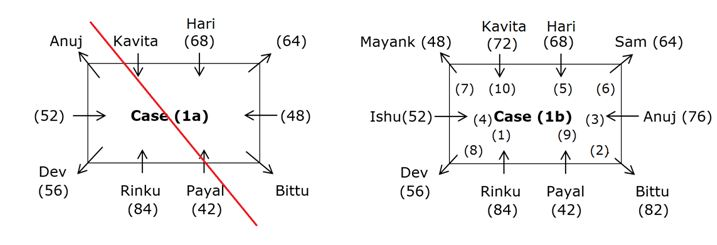Case (1a) is not valid as the one whose weight is 76kg sits facing Ishu and one person sits between Ishu and the one whose weight is 72kg.

Again after applying given condition of drawn cards we have final arrangement as follow: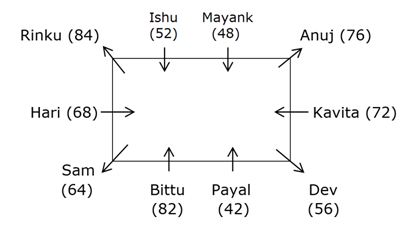Clearly, Bittu sits immediate left of Sam in the final arrangement.

Hence, option C is correct choice.

Clearly, only Payal remains unchanged after final arrangement.

Hence, option A is correct choice.

Clearly, only two person sits between the one whose age is 64 years and the one whose age is 52 years when counted right of the one whose age is 64 years.

Hence, option B is correct choice.

Directions (6-10):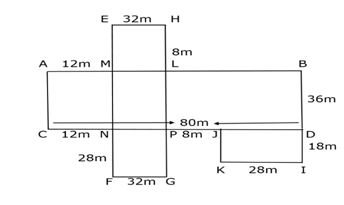We have:

• A, B, C and D are the corner points of the rectangular field in such a way that A is west of point B.
• C is south of point A, that means D is east of C.
• The length of largest side of field ABCD is 80m.
• Side CD is the largest side of the field, that means side AB = CD = 80m.
• E, F, G and H are the corner points of another rectangular field having area of 2304 m2 in such a way that Line EF intersects Line AB at M and Line CD at N respectively.
• C is 12m west of point N.
• Line GH intersects the rectangular field at L and P.
• H is 32m east of point E.

Area of EFGH = 2304m2.

EH = 32m

Thus, EF = 2304/32 = 72m.

Based on above given information we have: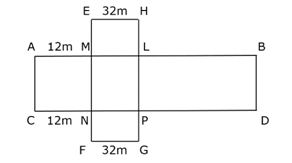Again, we have:

• D, I, J and K form another rectangular field in such a way that point J is 8m east of point P, that means PJ = 8m.

Thus, DJ = CD – (CN + PN + PJ)

DJ = 80m – (12m + 32m + 8m) = 28m.

• Area of the rectangular field DIJK is 504m2.

DI = 504/28 = 18m.

• Point I is 54m south of point B, that means BI = 54m.

Thus, BD = (54 – 18) = 36m.

• Point H is 8m from point L, that means HL = 8m.

PG = HG – (HL + LP)

PG = 72 – (8 + 36) = 28m.

Based on above given information we have final arrangement as follow: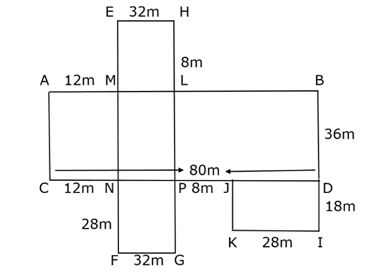Clearly, K is north-east of G.

GK = √(102 + 82) = 2√41m.

Hence, option A is correct choice.

Total distance covered in “FGPDB” = 132m.

Total distance covered in “FNCMB” = 144m.

Total distance covered in “FGLB” = 132m.

Total distance covered in “FEHLB” = 148m.

Hence, option E is correct choice.

Area of MLPN = (36 x 32) = 1152m2.

Area of EHLM = (32 x 8) = 256m2.

Thus, required difference = (1152 – 256) = 896m2.

Hence, option E is correct choice.

MH = √(322 + 82) = 4√68m.

Hence, option C is correct choice.